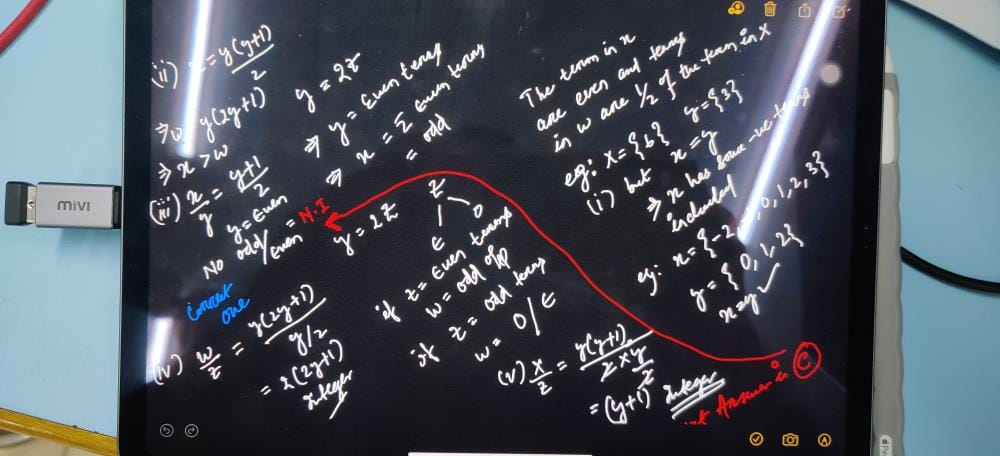x is the sum of y consecutive integers. w is ...

### Related Testx is the sum of y consecutive integers. w is the sum of z consecutive integers. If y = 2z, and y and z are both positive integers, then each of the following could be true EXCEPT
• a)
x = w
• b)
x > w
• c)
x/y is an integer
• d)
w/z is an integerPreitee Ranjan PradhanView courses related to this question Explore GMAT courses
 Explore GMAT coursesView courses related to this question1 Crore+ students have signed up on EduRev. Have you?

• ### A, B, C, D, E, and F are six consecutive positive odd integers in increasin... more(Scan QR code)